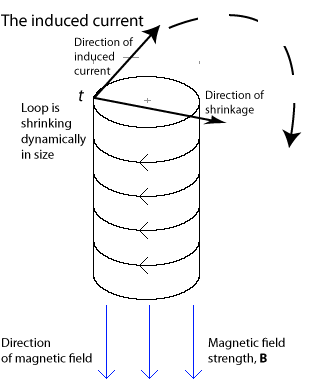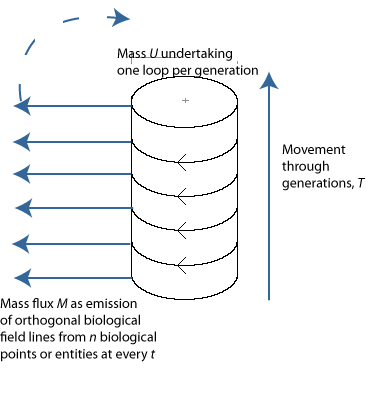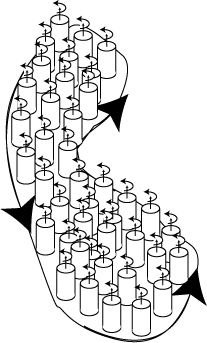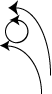# 37. Darwinian evolution as biological induction

The first step in handling a problem is to do what Darwin did, and describe it appropriately so it can be approached:

After returning from the Beagle voyage in October 1836, Darwin began arranging and cataloguing his specimens. In March of the next year he set down in his “Red Notebook” brief speculation on species change. … In September 1838 he read Malthus, which as he recalled, gave him “a theory by which to work”. The catalyst of Malthus precipitated out of Darwin’s thought the bare structure of his mechanism for natural selection. (Richards, 1992, p. 80)

What Darwin seems to have received intellectually, from his reading of Malthus, was the notion that a population-level phenomenon could be explained in an entirely non-interventionist way, using rational laws and explanations. Death rates were stable. They followed rational and fixed natural laws (Ariew, 2007).

Exactly when and what it was that congealed these ideas and moved Darwin so firmly away from a more traditional essentialist and Aristotelian viewpoint about the origin of species to the one now indelibly associated with his name is uncertain. Sandra Herbert writes in the above cited The Red Notebook of Charles Darwin that:

The whole run of entries on species in the second part of the Red Notebook is important for the limited purpose of dating specific passages …. It is also essential for the larger purpose of establishing a date for Darwin’s arrival at a belief in the mutability of species. On this last point it should be stated that while Darwin’s observations during the Beagle voyage were fundamental to his work on evolution, his notes from the voyage do not reveal him to have been an evolutionist. He was at the stage of asking basic questions. It should also be stated that there has previously been no fully satisfying evidence to document Darwin’s own claim that he began to form his views on the species question in ‘about’ March 1837. Undoubtedly the chief significance of the Red Notebook is that it provides such evidence. In the Red Notebook are found explicit indications that Darwin was ready to assert the possibility that “... one species does change into another ...” (Red Notebook, p. 130). Equally important, Darwin’s remarks on the species question in the second part of the notebook are sufficiently extended to allow one to characterize his position in some detail.

Darwin’s remarks on species in the second part of the notebook are directed towards three general topics: the geographical distribution of species, a comparison between the distribution of species through time and through space, and the generation of individuals and species. The central theoretical notion to emerge with respect to geographical distribution is that of the ‘representation of species’ (p. 130), or what Darwin referred to in his autobiography as ‘the manner in which closely allied animals replace one another in proceeding southwards over the [South American] Continent…’.From this notion Darwin drew the tentative conclusion that such representative species as (to take his example) the two South American rheas had descended from a common parent (p. 153e) (Herbert, 1980, p. 7).

Although we are now a lot closer to using our chosen instrument of a planimeter to measure natural selection and evolution then explain how biological mass can circulate from t to t over a given generation, and therefore also from one generation to another, we must first change the description of Darwin’s theory to make it more approachable.

We first note that material objects—resources in the environment—can become biological. This is in a very real sense to change their direction.

When a chemical component becomes encapsulated in a biological entity, it changes its trajectory. Something very different—at least, from our perspective—is happening to it. It follows a completely different path from the one it would otherwise have pursued. It is therefore moving in a completely different direction than if it had not been so incorporated. This change in direction establishes a vector. We can then further say that Darwin’s essential claim is that a change in the numbers of any given set of those biological entities that are capable of instigating such changes in direction, within the material world, will also and necessarily engage in some form of transmissible and inheritable changes amongst themselves as they similarly induce their successors to themselves to do the same as they have done. If we now call these instigations and changes of direction of materials ‘fluxes’; and if we then call the transmissibilities and the engagements of successors ‘biological induction’, then we can immediately conquer natural selection and evolution with vectors. We simply proceed as follows ….

Figure 34: Biological Induction: The Heritability of Darwinian EvolutionIn 1831 Michael Faraday discovered, as in Figure 34, that a changing magnetic field—which is a change in magnetic flux—induces an electric field, which can be thought of as flowing along point-current-elements, dl (Cooper, 1968). Thus at the time-point t at the head of the two arrows, the diameter of a magnetic loop located within a given magnetic field, B, and that is pointing down the page, is being actively decreased, and so directly along the page and rightwards towards the margin.

The standard electrostatic fields generated, as in Figure 19, by point-electric charges diverge, as we have seen, from positive to negative. In complete contrast to those, the electrical fields that Faraday induced by moving a magnet emulated magnetic behaviour by looping from point to point and along their current elements, and as in Figure 33 for the Biot-Savart law. They are thus induced in, and are reciprocally affected by, the electrical and the magnetic potentials, capabilities, and behaviours of all points upon whatever material generates and/or conducts them with its deformation or movement.

Figure 35: The Temporal Continuity of the GenerationsBy Fleming’s right-hand rule, and as in Figure 34, Faraday’s induced electric current will now flow directly into the page from its point of induction at t, and be induced directly along whatever loop is being manipuated (Cooper, 1968, p. 210, 492). Similar induced currents will therefore flow from point to point all around the loop as it continues to shrink. And since the loop is continuous, then these currents can clearly “change levels” as they loop around and such that a given angle of emission is eventually repeated. These inductions are clearly ‘inheritable’—in the strict Darwinian sense—in that each level of induction represents a generation, and—as in Figure 34 and Figure 35—they are clearly transmitted. If the strength of the magnetic field is B then the magnitude of this induced current depends entirely upon B/t, which is the time-rate at which the loop is being decreased at every t through that magnetic field. The German physicist Heinrich Lenz later demonstrated that this induced field always opposes the change in flux. Therefore, the induced field always strives to restore the system.

Faraday’s discovery was against all contemporary theory. It went unexplained for a generation. It was the puzzle that Maxwell, after having first written to Thomson, set out to resolve.

As in Figure 35, Faraday’s magnetically based induction bears a most remarkable similarity to Darwin’s biological induction as we have now characterized it, and complete with the passage of “traits” and chemical components across the generations.

Figure 36: Divergence and flux densityOne of Maxwell’s important discoveries in analysing the Faraday effect was that divergence is a measure of the flux density. As in Figure 36, flux can be conceived of as passing through an infinitesimally small unit sphere. Divergence is then the net flux per unit volume. When a flux density increases, the flux source or sink has also increased in its magnitude. And since divergence = flux/volume, then flux and volume are inversely related. The smaller is the count or volume through which a given flux passes, then the greater is the divergence.

Although Maxwell went through a variety of names, his other important discovery was what he eventually called the ‘curl’ of a vector field:

(1) The result of applied to a scalar function might be called the slope of the function. Lamé might call it the differential parameter, but the thing itself is a vector, now slope is a vector word, whereas parameter has, to say the least, a scalar sound.

(2) If the original function is a vector then applied to it may give two parts. The scalar part I would call the Convergence of the vector function and the vector part I would call the twist of the vector function.

(Here the twist has nothing to do with a screw or helix. If the words turn or version would do they would be better than twist for twist suggests screw.)

Twirl is free from the screw notion and is sufficiently racy. Perhaps it is too dynamical for pure mathematicians so for Cayleys sake I might say Curl (after the fashion of Scroll).

Hence the effect of on a scalar function is to give the slope of that scalar and its effect on a vector function is to give the convergence and the twirl of that vector.

The result of 2 applied to any function may be called the concentration of that function because it indicates the mode in which the value of the function at a given point exceeds (in the Hamiltonian sense) the average value of the function in a little spherical surface drawn round it.

Now if σ be a vector function of ρ and F a scalar function of ρ

F is the slope of F

VF is the twirl of the slope which is necessarily zero

SF = 2F is the convergence of the slope, which is the concentration of F.

Also Sσ is the convergence of σ

Vσ is the twirl of σ.

Now the convergence being a scalar if we operate on it with we find that it has a slope but no twirl.

Hence 2σ, the concentration of σ is the slope of the convergence of σ together with the twirl of the twirl of s & ∴ the sum of two vectors (Maxwell, 1870, pp. 568–569).

Had Darwin but realized it; and had he understood the implications; then the above Maxwell discoveries in the vector calculus would have given him everything he needed to describe his natural selection.

Maxwell has stated all the main identities and definitions of the vector calculus, and which we can put to good use for our own purposes. A vector curl is the tendency to induce the attributes of a field to move or swirl about the points contained within the field, much like water swirls about a plug hole as a sink drains. Along with that curl goes a vector circulation which is the total curling force emanating all about the closed path or boundary surrounding those points. This circulation is the sum of all the forces about all the points concerned in forming that boundary—which could, for example, be molecules. Each such point is characterized by a suitable curl about itself—which is effectively a circulation density attributable to each—and that contributes to the overall circulation about their common boundary.

Figure 37: Circulation and curlBy Stokes’ theorem—one of the four important theorems in the vector calculus—no field lines with zero curl, such as exists in either a gravitational or an electrostatic field, can form a closed loop. As in Figure 37, if a field can form a boundary with a closed loop, then a vector curl—which is a vector cross-product symbolized by  x—exists about every point, making them all spin in a vertical sense about their axes. And by the same token, if there is no curl about the points of the field, then there is no circulation about the boundary.

If we now reconsider the infinitely small sphere of Figure 36 depicting divergence, then the flux will also swirl and circulate about the sphere’s boundary as it enters. The curl is in this case a measure of the intensity or density of this swirl of flux about a given point of interest. The curl is the circulation evidenced per unit area of the sphere’s surface. Thus if the sphere shrinks in size as a given quantity of flux enters, the divergence increases as the volume decreases … and the curl also increases because the circulation per unit surface area immediately increases in that the same circulation must now pass over a smaller area.

To return to flux and divergence, Green’s theorem relating the two can be stated for a plane figure. If C is a piecewise smooth curve—i.e. is a finite union of smooth curves that intersect only on their boundaries—and encloses a simply-connected region R where F = F1i + F2j is a vector field and such that F1 and F2 each have continuous first partial derivatives within an open region containing R, then:

CFn ds = C F1 dy - F2 dx = R div F dA = R F dA

where each of the two single integrals on the left and set equal to each other are each the outward flux, while the two double integrals set equal to each other and upon their right are the divergence.

Figure 38: Two-Dimensional CurlAnd although a curl is most usually known in three dimensions, such as in electromagnetics where it operates through the right-hand rule (so for the vector cross-product c, then if the thumb and index finger point in the directions of a and b respectively, the middle finger will point in the direction of travel for c), circulation and curl can also exist in the plane as in:

CFT ds = C F1 dx + F2 dy = R curl F dA = R (F2/x - F1/y) dA

where T is again the unit tangent vector and where the sum of the two partial differentials on the far right, F2/x - F1/y, is the two-dimensional curl or the circulation per unit area. If the force on the field acts so the flow or action it causes diverges so the net flow is faster on one field line than it is on another, then as in Figure 38, that variation will be imposed on each point through that difference on either side. That difference in rate of flow either side then causes the curl. And … these are the phenomena we need to explain natural selection and to account completely for Darwinian competition and evolution, and to create a quantum biology.